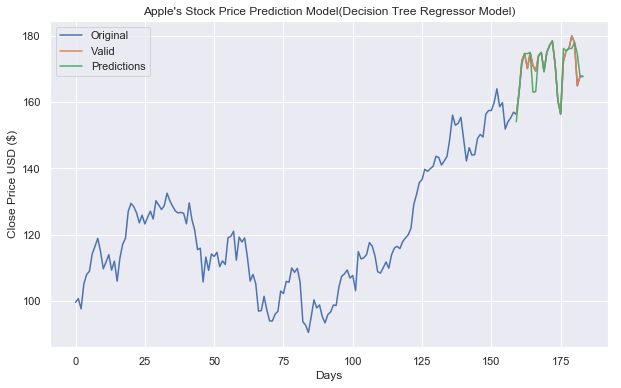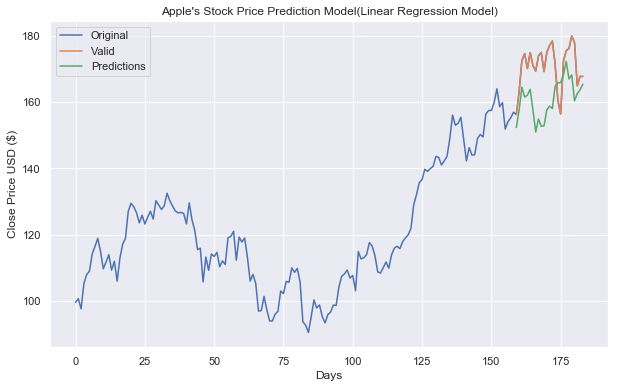• +91-9872993883
• +91-8283824812
• info@ris-ai.com

# Stock Price Prediction with Machine Learning ¶

The Stock Market is known as a place where people can make a fortune if they can successfully predict stock prices as Stock Price Prediction is very important in finance and business for decision making. In this article , we will create a Linear Regression model and a Decision Tree Regression Model to Predict Apple’s Stock Price using Machine Learning and Python . Stock Dataset is also provided , you can down load it.

A stock price may depend on several factors operating in the current world and stock market. We will try to take into account a combination of mainly two factors:

• 1. How the increase and decrease of stock prices of the other companies affect the stock price of a given target company.
• 2. The past performances of the target company.

Import pandas to import a CSV file:

In :
import pandas as pd

         Date        Open        High         Low       Close   Adj Close  \
0  2014-09-29  100.589996  100.690002   98.040001   99.620003   93.514290
1  2014-10-06   99.949997  102.379997   98.309998  100.730003   94.556244
2  2014-10-13  101.330002  101.779999   95.180000   97.669998   91.683792
3  2014-10-20   98.320000  105.489998   98.220001  105.220001   98.771042
4  2014-10-27  104.849998  108.040001  104.699997  108.000000  101.380676

Volume
0  142718700
1  280258200
2  358539800
3  358532900
4  220230600


To get the number of training days:

In :
print("training days =",apple.shape)

training days = (184, 7)


To Visualize the close price Data:

In :
import matplotlib.pyplot as plt
import seaborn as sns
sns.set()
plt.figure(figsize=(10, 4))
plt.title("Apple's Stock Price")
plt.xlabel("Days")
plt.ylabel("Close Price USD ($)") plt.plot(apple["Close"]) plt.show()To get the close price: In : apple = apple[["Close"]] print(apple.head())   Close 0 99.620003 1 100.730003 2 97.669998 3 105.220001 4 108.000000  Creating a variable to predict ‘X’ days in the future: In : futureDays = 25  Create a new target column shifted ‘X’ units/days up: In : apple["Prediction"] = apple[["Close"]].shift(-futureDays) print(apple.head()) print(apple.tail())   Close Prediction 0 99.620003 123.250000 1 100.730003 125.320000 2 97.669998 127.099998 3 105.220001 124.750000 4 108.000000 130.279999 Close Prediction 179 179.979996 NaN 180 178.020004 NaN 181 164.940002 NaN 182 167.779999 NaN 183 167.779999 NaN  To create a feature dataset (x) and convert into a numpy array and remove last ‘x’ rows/days: In : import numpy as np x = np.array(apple.drop(["Prediction"], 1))[:-futureDays] # print(x)  To create a target dataset (y) and convert it to a numpy array and get all of the target values except the last ‘x’ rows days: In : y = np.array(apple["Prediction"])[:-futureDays] print(y)  [123.25 125.32 127.099998 124.75 130.279999 128.949997 127.620003 128.770004 132.539993 130.279999 128.649994 127.169998 126.599998 126.75 126.440002 123.279999 129.619995 124.5 121.300003 115.519997 115.959999 105.760002 113.290001 109.269997 114.209999 113.449997 114.709999 110.379997 112.120003 111.040001 119.080002 119.5 121.059998 112.339996 119.300003 117.809998 119.029999 113.18 106.029999 108.029999 105.260002 96.959999 97.129997 101.419998 97.339996 94.019997 93.989998 96.040001 96.910004 103.010002 102.260002 105.919998 105.669998 109.989998 108.660004 109.849998 105.68 93.739998 92.720001 90.519997 95.220001 100.349998 97.919998 98.830002 95.330002 93.400002 95.889999 96.68 98.779999 98.660004 104.209999 107.480003 108.18 109.360001 106.940002 107.730003 103.129997 114.919998 112.709999 113.050003 114.059998 117.629997 116.599998 113.720001 108.839996 108.43 110.059998 111.790001 109.900002 113.949997 115.970001 116.519997 115.82 117.910004 119.040001 120. 121.949997 129.080002 132.119995 135.720001 136.660004 139.779999 139.139999 139.990005 140.639999 143.660004 143.339996 141.050003 142.270004 143.649994 148.960007 156.100006 153.059998 153.610001 155.449997 148.979996 142.270004 146.279999 144.020004 144.179993 149.039993 150.270004 149.5 156.389999 157.479996 157.5 159.860001 164.050003 158.630005 159.880005 151.889999 154.119995 155.300003 156.990005 156.25 163.050003 172.5 174.669998 170.149994 174.970001 171.050003 169.369995 173.970001 175.009995 169.229996 175. 177.089996 178.460007 171.509995 160.5 156.410004 172.429993 175.5 176.210007 179.979996 178.020004 164.940002 167.779999 167.779999]  ### Split the data into 75% training and 25% testing ¶ In : from sklearn.model_selection import train_test_split xtrain, xtest, ytrain, ytest = train_test_split(x, y, test_size=0.25)  ### Creating Models¶ In : # Creating the decision tree regressor model from sklearn.tree import DecisionTreeRegressor tree = DecisionTreeRegressor().fit(xtrain, ytrain) # creating the Linear Regression model from sklearn.linear_model import LinearRegression linear = LinearRegression().fit(xtrain, ytrain)  To get the last ‘x’ rows/days of the feature dataset: In : xfuture = apple.drop(["Prediction"], 1)[:-futureDays] xfuture = xfuture.tail(futureDays) xfuture = np.array(xfuture) print(xfuture)  [[143.649994] [148.960007] [156.100006] [153.059998] [153.610001] [155.449997] [148.979996] [142.270004] [146.279999] [144.020004] [144.179993] [149.039993] [150.270004] [149.5 ] [156.389999] [157.479996] [157.5 ] [159.860001] [164.050003] [158.630005] [159.880005] [151.889999] [154.119995] [155.300003] [156.990005]]  #### To see the model tree prediction¶ In : treePrediction = tree.predict(xfuture) print("Decision Tree prediction =",treePrediction)  Decision Tree prediction = [154.119995 163.050003 171.509995 174.669998 174.669998 174.970001 163.050003 163.18 173.970001 175.009995 169.229996 175. 177.089996 178.460007 171.509995 160.5 156.410004 176.210007 175.5 176.210007 176.210007 178.020004 174.669998 167.779999 167.779999]  #### To see the model linear regression prediction ¶ In : linearPrediction = linear.predict(xfuture) print("Linear regression Prediction =",linearPrediction)  Linear regression Prediction = [152.36331808 157.55282578 164.53078983 161.55977169 162.09729294 163.89553212 157.57236115 151.01464554 154.93363781 152.72493108 152.8812893 157.63099658 158.83309374 158.08056419 164.81420173 165.87946237 165.8990124 168.2054556 172.3003698 167.0033731 168.22500563 160.41632462 162.59571315 163.74894208 165.40059121]  ## Visualize decision tree predictions¶ In : predictions = treePrediction valid = apple[x.shape:] valid["Predictions"] = predictions plt.figure(figsize=(10, 6)) plt.title("Apple's Stock Price Prediction Model(Decision Tree Regressor Model)") plt.xlabel("Days") plt.ylabel("Close Price USD ($)")
plt.plot(apple["Close"])
plt.plot(valid[["Close", "Predictions"]])
plt.legend(["Original", "Valid", "Predictions"])
plt.show()

/.local/lib/python3.5/site-packages/ipykernel_launcher.py:3: SettingWithCopyWarning:
A value is trying to be set on a copy of a slice from a DataFrame.
Try using .loc[row_indexer,col_indexer] = value instead

See the caveats in the documentation: http://pandas.pydata.org/pandas-docs/stable/indexing.html#indexing-view-versus-copy
This is separate from the ipykernel package so we can avoid doing imports until## Visualize the linear model predictions¶

In :
predictions = linearPrediction
valid = apple[x.shape:]
valid["Predictions"] = predictions
plt.figure(figsize=(10, 6))
plt.title("Apple's Stock Price Prediction Model(Linear Regression Model)")
plt.xlabel("Days")
plt.ylabel("Close Price USD (\$)")
plt.plot(apple["Close"])
plt.plot(valid[["Close", "Predictions"]])
plt.legend(["Original", "Valid", "Predictions"])
plt.show()

/.local/lib/python3.5/site-packages/ipykernel_launcher.py:3: SettingWithCopyWarning:
A value is trying to be set on a copy of a slice from a DataFrame.
Try using .loc[row_indexer,col_indexer] = value instead

See the caveats in the documentation: http://pandas.pydata.org/pandas-docs/stable/indexing.html#indexing-view-versus-copy
This is separate from the ipykernel package so we can avoid doing imports until